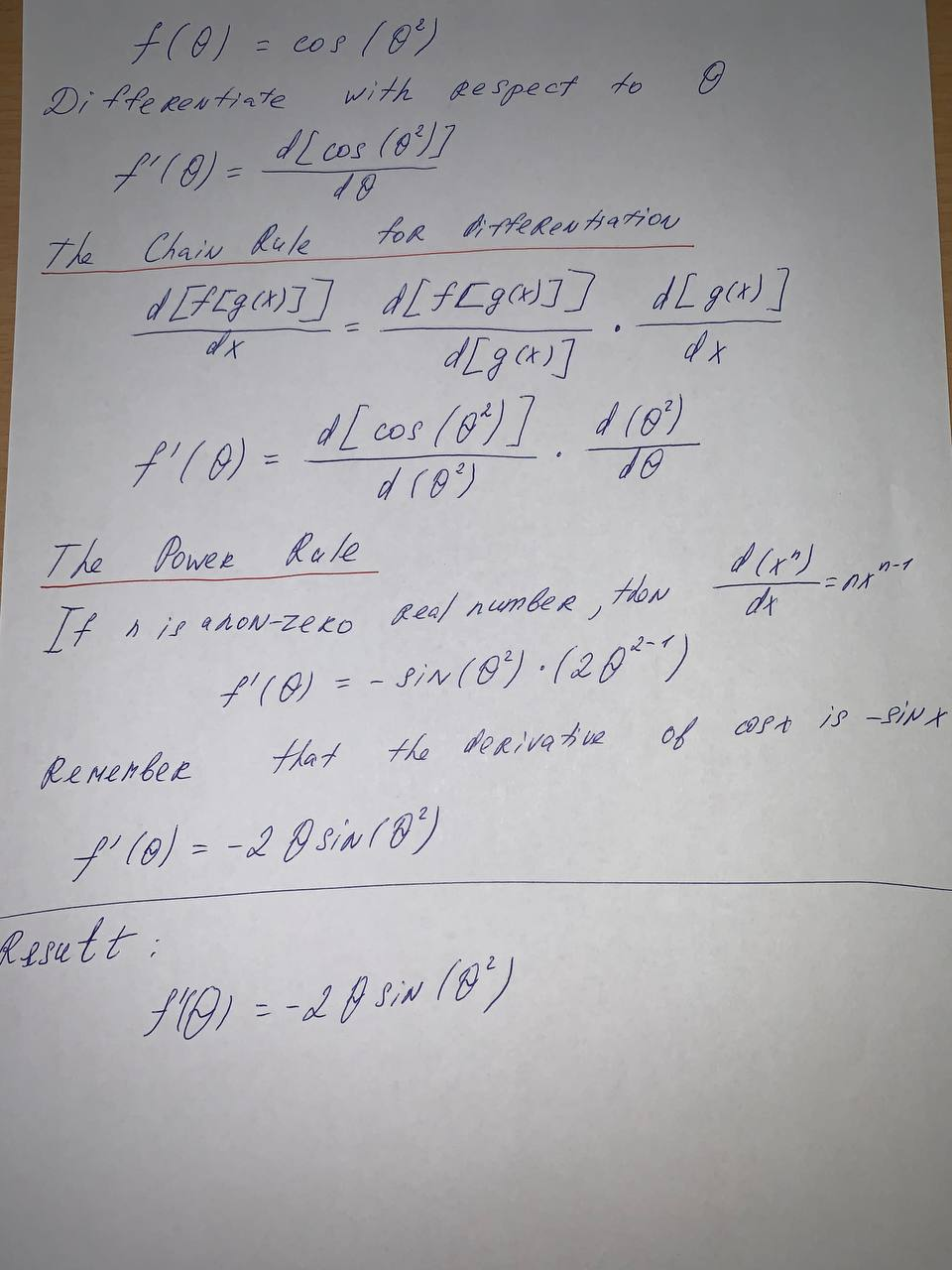#### Didn’t find what you are looking for?

Question# Find the derivative of the function. f(\theta) = \cos(\theta^2)

Derivatives
ANSWEREDFind the derivative of the function.
$$f(\theta) = \cos(\theta^2)$$